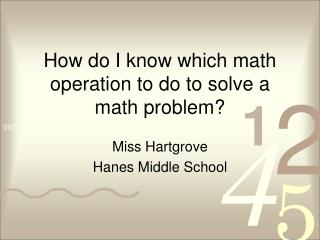DownloadDownload PresentationHow do I know which math operation to do to solve a math problem?

# How do I know which math operation to do to solve a math problem?

Download Presentation## How do I know which math operation to do to solve a math problem?

- - - - - - - - - - - - - - - - - - - - - - - - - - - E N D - - - - - - - - - - - - - - - - - - - - - - - - - - -
##### Presentation Transcript

1. How do I know which math operation to do to solve a math problem? Miss Hartgrove Hanes Middle School

2. Lesson Outline Today we are going to: • Review the Four Step Plan to Solve Math Problems • Identify the four math operations • Identify clue words that tell us which math operation we need to use.

3. Review Yesterday we talked about the four step plan to solving math problems. To help us review the steps to solve math problems, you are going to do a matching game in your small groups. Look at your work system and get out your blue folder. You should work in your small groups to put the steps in order.

4. What is a math operation? • Addition • Subtraction • Multiplication • Division

5. When you are asked to identify a math operation, you are being asked if you should add, subtract, multiply, or divide!

6. Why do we need to learn math clue words? Word problems do not specifically tell us to add, subtract, multiply, or divide. We need to know the clue words so we can determine if we should add, subtract, multiply, or divide.

7. I should add when I see… • Sum • Total • Increased by • Together • Altogether • Both • In all

8. I should subtract when I see… • Decreased by • Minus • Difference • How many more • How much more • Take away

9. I should multiply when I see… • Of • Times • Product of

10. I should divide when I see… • Per • Quotient of

11. Let’s practice Using the multiple choice cards, you should work with your group to identify the math operation that matches the clue word.

12. How many more • Addition • Subtraction • Multiplication • Division

13. Per • Addition • Subtraction • Multiplication • Division

14. Product of • Addition • Subtraction • Multiplication • Division

15. altogether • Addition • Subtraction • Multiplication • Division

16. Let’s Practice To see how many of the clue words you remember, you are going to complete a fill-in-the-blank activity. The fill-in-the-blank activity is in your yellow folder. The completed activity will be used as a resource for you as we solve math problems throughout the year.

17. Match Time! You are going to work with your small group to complete a matching activity. In the envelope you will find two sets of cards. Some of the cards will have math operations written on them. Other cards will have sentences from word problems that contain some of the clue words. You are going to match the clue words to the math operation.